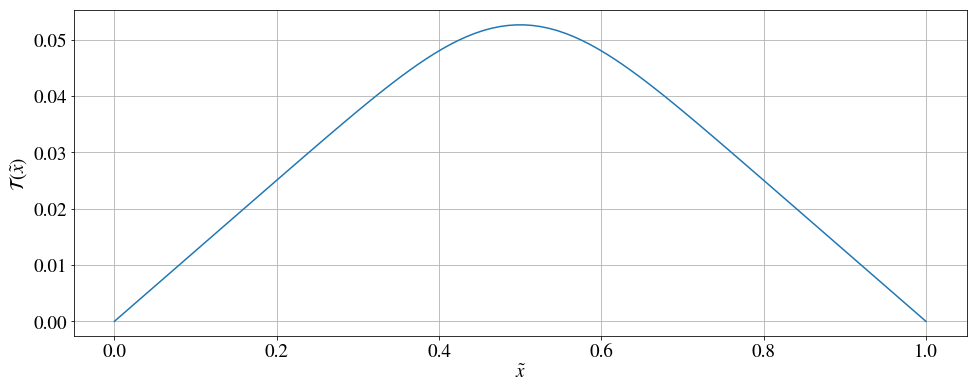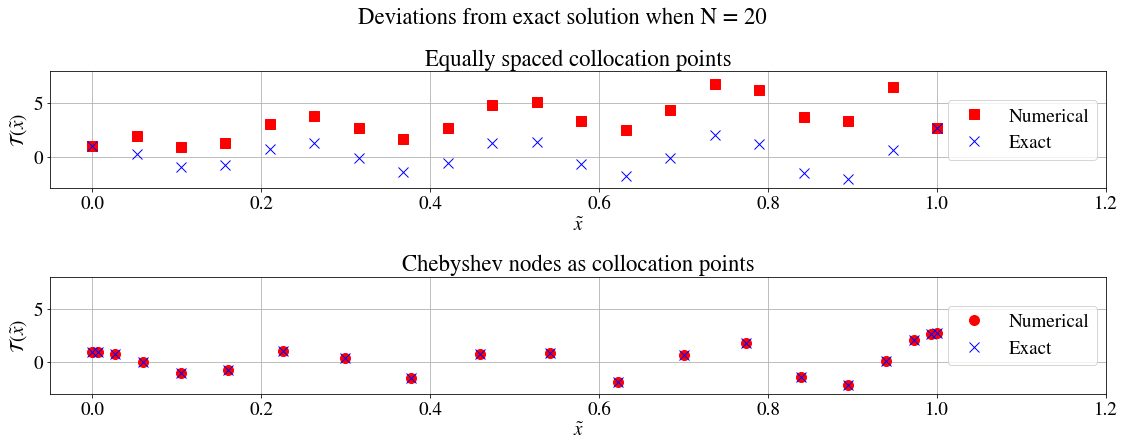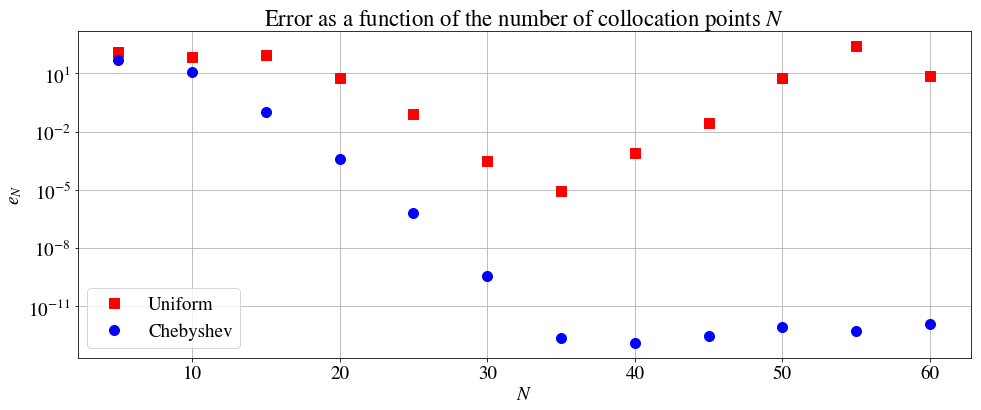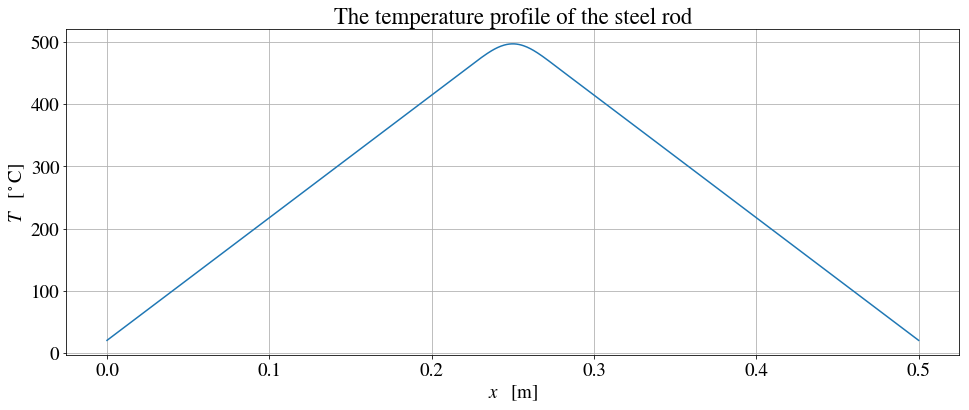# One-dimensional stationary heat equation¶

### Examples - Thermodynamics¶

Last edited: March 16th 2018

This notebook is based on an assignment given in the course TMA4320 Introduction to Scientific Computation at NTNU, spring 2017.

## Introduction¶

The heat equation is a partial differential equation that describes the distribution of heat in a system. It can be solved in order to find the temperature variations in a physical body. In this notebook, we shall first derive the heat equation describing a system containing a heat source. We will be focusing on the steady state solution, when the system has stabilised and there is no time dependence. As a concrete example, we will be looking at a thin rod with fixed temperatures at the ends. The rod is thin enough to be regarded as one-dimensional and is heated by a Gaussian heat source. The stationary heat equation will be solved using a collocation method, approximating the solution as a sum of Lagrange polynomials.

## A non-rigorous derivation of the heat equation¶

Consider a uniform system of fixed volume $\mathcal{V}$ and no exchange of particles with the environment.

Let $u(\mathbf{r}, t)$ denote the energy density of the system, $\mathbf{j}(\mathbf{r}, t)$ the heat flux going out of the system into the environment and let $\dot{q}(\mathbf{r}, t)$ denote the heat that enters the system from the heat source per unit volume per unit time (heating rate per unit volume). The change in the total energy of the system must be equal to the energy entering the system from the heat source minus the energy that flows out of the system, hence

\begin{align*} \frac{\mathrm{d}}{\mathrm{d}t} \int_\mathcal{V} u(\mathbf{r}, t) \,\mathrm{d}V &= \int_\mathcal{V} \dot{q}(\mathbf{r}, t) \,\mathrm{d}V - \oint_{\partial\mathcal{V}} \mathbf{j}(\mathbf{r}, t) \cdot \mathrm{d}\mathbf{S} \\ \int_\mathcal{V} \frac{\mathrm{\partial}}{\mathrm{\partial}t} u(\mathbf{r}, t) \,\mathrm{d}V &= \int_\mathcal{V} \dot{q}(\mathbf{r}, t) \,\mathrm{d}V - \int_\mathcal{V} \nabla\cdot \mathbf{j}(\mathbf{r}, t) \,\mathrm{d}V \\ \int_\mathcal{V} \left[ \frac{\mathrm{\partial}}{\mathrm{\partial}t} u(\mathbf{r}, t) + \nabla\cdot \mathbf{j}(\mathbf{r}, t) - \dot{q}(\mathbf{r}, t) \right] \,\mathrm{d}V &= 0 \end{align*}$$\implies \frac{\mathrm{\partial}}{\mathrm{\partial}t} u(\mathbf{r}, t) + \nabla\cdot \mathbf{j}(\mathbf{r}, t) - \dot{q}(\mathbf{r}, t) = 0 \quad .$$

We have assumed that the volume of the system is constant, which implies that there is no pressure-volume work involved. The first law of thermodynamics then reads

\begin{align*} \mathrm{d}U &= \mathrm{d}Q \\ &= C_V \mathrm{d}T \quad , \end{align*}

where $U$ is the internal energy, $T$ the temperature of the system and $C_V$ the heat capacity of the system under constant volume. The partial time derivative of the internal energy density $u$ can thus be expressed as

$$\frac{\mathrm{\partial}u}{\mathrm{\partial}t} = \rho c_V \frac{\mathrm{\partial}T}{\mathrm{\partial}t} \quad .$$

Here, $c_V$ is the heat capacity per unit mass and $\rho$ is the mass density of the system.

Fourier's law is an empirical law that states that the heat flux is proportional to the negative temperature gradient (heat flows from regions of higher temperature to regions of lower temperature), $$\mathbf{j} = - \kappa \, \nabla T \quad .$$

The constant $\kappa$ is called the thermal conductivity.

Inserting these two expressions into the energy conservation equation yields the heat equation with a source term

$$\frac{\mathrm{\partial}}{\mathrm{\partial}t} T(\mathbf{r}, t) = \frac{\kappa}{\rho c_V} \nabla^2 T(\mathbf{r}, t) + \frac{1}{\rho c_V} \, \dot{q}(\mathbf{r}, t) \quad .$$

We are interested in the stationary solution and no explicit time dependence implies that

\begin{align*} 0 &= \frac{\kappa}{\rho c_V} \nabla^2 T(\mathbf{r}) + \frac{1}{\rho c_V} \, \dot{q}(\mathbf{r}) \\ -\nabla^2 T(\mathbf{r}) &= \frac{1}{\kappa} \, \dot{q}(\mathbf{r}) \quad . \end{align*}

In one dimension this becomes

$$-\frac{\mathrm{d}^2}{\mathrm{d}x^2} T(x) = \frac{1}{\kappa} \, \dot{q}(x) \quad .$$

The SI units of the relevant physical quantities can be found using dimensional analysis:

We know that $[T] = \mathrm{K}$ and $[\dot{q}] = \mathrm{Jm^{-3}s^{-1}}$, and wish to find the unit of $\kappa$. From the one-dimensional heat equation we have that

\begin{align*} [\kappa] &= [\dot{q}(x)]\, \cdot \left[\frac{\mathrm{d}^2}{\mathrm{d}x^2} T(x)\right]^{-1} \\ &= \mathrm{Jm^{-3}s^{-1}} \cdot (\mathrm{K m^{-2}})^{-1} \\ &= \mathrm{JK^{-1}m^{-1}s^{-1}} \quad . \end{align*}

## Example: Modelling a thin metal rod that is heated by a candle¶

Consider a thin metal rod of length $L = b - a\,$ and cross-sectional area $A$, which are both assumed to be constant. The rod is heated by a burning candle that is modelled by a Gaussian heat source

$$\dot{q}(x) = \dot{q}_0 \exp\left[-\frac{1}{2}\left(\frac{x-x_0}{\sigma}\right)^2\right] \quad ,$$

where $\dot{q}_0$ is related to the power of the heat source $P_\mathrm{src}$, $A$ and $\sigma$, which will be explained later in this notebook. A pair of heat reservoirs of constant temperatures $T(a)$ and $T(b)$ keep the temperature of the rod fixed at the ends. See Figure 1.Figure 1: A sketch depicting the physical situation. The ends of the metal rod are attached to heat reservoirs of temperatures $T(a)$ and $T(b)$.

We start by rewriting the heat equation into a more numerically tractable form

\begin{align*} -\frac{\mathrm{d}^2}{\mathrm{d}x^2} T(x) &= \frac{\dot{q}_0}{\kappa} \exp\left[-\frac{1}{2}\left(\frac{x-x_0}{\sigma}\right)^2\right] \\ -\frac{\mathrm{d}^2}{\mathrm{d}x^2} \left[\frac{\kappa \, T(x)}{\dot{q}_0}\right] &= \exp\left[-\frac{1}{2}\left(\frac{x-x_0}{\sigma}\right)^2\right] \quad . \end{align*}

We also introduce the dimensionless variables $\tilde{x} = (x-a)~/~L$ and $\tilde{\sigma} = \sigma~/~L$ mapping $a \mapsto 0$ and $b \mapsto 1$. This yields the following dimensionless equation

\begin{align*} -\frac{\mathrm{d}^2}{\mathrm{d}\tilde{x}^2} \left[\frac{\kappa \, T(\tilde{x})}{L^2 \, \dot{q_0}}\right] &= \exp\left[-\frac{1}{2}\left(\frac{\tilde{x}-\tilde{x}_0}{\tilde{\sigma}}\right)^2\right] \\ -\frac{\mathrm{d}^2}{\mathrm{d}\tilde{x}^2} \mathcal{T}(\tilde{x}) &= f(\tilde{x} ; \tilde{x}_0, \tilde{\sigma}) \quad . \end{align*}

### Expanding the solution in a basis of Lagrange polynomials¶

The dimensionless heat equation can be solved by discretising the interval $[0,1]$ on the $\tilde{x}$-axis and approximating the solution on the whole domain as a sum of Lagrange polynomials, demanding that the approximation satisfies the heat equation at each discrete point. The points on the domain, at which the approximate solution satisfies the heat equation, are called collocation points. For a short introduction to Lagrange interpolation, please have a look at the relevant section in our notebook on polynomial interpolation. The interval $[0,1]$ is discretised into $N$ points, $0 = \tilde{x}_0 \lt \tilde{x}_1 \lt \dots \lt \tilde{x}_{N-2} \lt \tilde{x}_{N-1} = 1$. The solution is approximated by

$$\mathcal{T}(\tilde{x}) = \sum_{i = 0}^{N-1} c_i \mathrm{L}_i(\tilde{x}) \quad ,$$

where $$\mathrm{L}_i(\tilde{x}) \equiv \prod_{k = 0}^{N-1} \frac{\tilde{x} - \tilde{x}_k}{\tilde{x}_i - \tilde{x}_k}$$

is the Lagrange polynomial corresponding to the point $\tilde{x}_i$. The Lagrange polynomials have the important property $\mathrm{L}_i(\tilde{x}_j) = \delta_{ij}$. Inserting the polynomial expansion into the heat equation and applying this property yields

\begin{cases} c_0 &= \mathcal{T}_a \\ -\sum_{j = 0}^{N-1} c_j \mathrm{L}_j''(\tilde{x}_i) &= f(\tilde{x}_i), & i = 1, \cdots, N-2 \\ c_{N-1} &= \mathcal{T}_b \end{cases}

This system of linear equations can be written in matrix form $A\vec{c} = \vec{f}$ :

$$\begin{pmatrix} 1 & 0 & \cdots & 0 \\ -\mathrm{L}_0''(\tilde{x}_1) & -\mathrm{L}_1''(\tilde{x}_1) & \cdots & -\mathrm{L}_{N-1}''(\tilde{x}_1) \\ -\mathrm{L}_0''(\tilde{x}_2) & -\mathrm{L}_1''(\tilde{x}_2) & \cdots & -\mathrm{L}_{N-1}''(\tilde{x}_2) \\ \vdots & \vdots & \ddots & \vdots \\ -\mathrm{L}_0''(\tilde{x}_{N-2}) & -\mathrm{L}_1''(\tilde{x}_{N-2}) & \cdots & -\mathrm{L}_{N-1}''(\tilde{x}_{N-2}) \\ 0 & 0 & \cdots & 1 \end{pmatrix} \begin{pmatrix} c_0 \\ c_1 \\ c_2 \\ \vdots \\ c_{N-2} \\ c_{N-1} \end{pmatrix} = \begin{pmatrix} \mathcal{T}_a \\ f(\tilde{x}_1) \\ f(\tilde{x}_2) \\ \vdots \\ f(\tilde{x}_{N-2}) \\ \mathcal{T}_b \end{pmatrix} \quad .$$

Let $X = \{\tilde{x}_0, \tilde{x}_1, \cdots, \tilde{x}_{N-1}\}$. The second derivative of the Lagrange polynomials can then be expressed by

$$\mathrm{L}_i''(\tilde{x}) = \sum_{\substack{x' \in X \setminus \{\tilde{x}_i\} \\ x'' \in X \setminus \{\tilde{x}_i, x'\}}} \frac{\mathrm{L}_i(\tilde{x})}{(\tilde{x} - x')(\tilde{x} - x'')} \quad .$$

This expression gets easier to evaluate numerically when one only considers $\tilde{x} \in X$. In this case, the expression can be rewritten to avoid division by zero.

When $i = j$,

$$\mathrm{L}_i''(\tilde{x}_i) = \sum_{\substack{x' \in X \setminus \{\tilde{x}_i\} \\ x'' \in X \setminus \{\tilde{x}_i, x'\}}} \frac{2}{(\tilde{x}_i - x')(\tilde{x}_i - x'')} \quad .$$

When $i \neq j$,

$$\mathrm{L}_i''(\tilde{x}_j) = \frac{2}{\tilde{x}_i - \tilde{x}_j} \prod_{\, x' \in X \setminus \{ \tilde{x}_i, \tilde{x}_j \}} \left(\frac{\tilde{x}_j - x'}{\tilde{x}_i - x'}\right) \sum_{x'' \in X \setminus \{ \tilde{x}_i, \tilde{x}_j \}} \frac{1}{\tilde{x}_j - x''} \quad .$$

Deriving the expression for the second derivative of the Lagrange polynomials is left as an exercise for the reader (Hint: logarithmic differentiation).

The heat equation is solved by finding the coefficients of the Lagrange polynomials, which is done by solving the system of linear equations that is described above.

### Implementing and verifying the solver¶

Now it is time to write some code. We begin by implementing functions that evaluate the Lagrange polynomials and their second derivative, as well as functions that solve the system of linear equations and the heat equation.

In :
%matplotlib inline
import numpy as np
import matplotlib.pyplot as plt
import itertools
from scipy.integrate import simps
import scipy.linalg.lapack as lapack

# Set some figure parameters
newparams = {
'figure.figsize': (16, 6), 'axes.grid': True,
'lines.linewidth': 1.5, 'font.size': 19, 'lines.markersize' : 10,
'mathtext.fontset': 'stix', 'font.family': 'STIXGeneral'}
plt.rcParams.update(newparams)

def lagrangepoly(xarr, i, x):
""" Evaluates the Lagrange polynomial L_i(x).

Parameters:
xarr: float array. Contains the points that define the particular set of Lagrange polynomials.
i: int (positive). Selects the Lagrange polynomial corresponding to the point xarr[i].
x: float. The point at which to evaluate the polynomial.

Returns: The value of the Lagrange polynomial evaluated at x.
"""
xs = np.delete(xarr, i)
return np.prod(x - xs) / np.prod(xarr[i] - xs)

def lagrangepoly_2nd_deriv(xarr, i, j):
""" Computes the second derivative of the Lagrange polynomial, L''_i(x_j).
This function is limited to evaluating the second derivative at points contained in xarr only.

Parameters:
xarr: float array. Contains the points that define the particular set of Lagrange polynomials.
i: int (positive). Selects the Lagrange polynomial corresponding to the point xarr[i].
j: int (positive). Selects the point xarr[j] at which to evaluate the second derivative.

Returns: The value of the second derivative of the Lagrange polynomial evaluated at xarr[j].
"""
res = 0.
if i == j:
# Exclude element x[i] to avoid zero division
xs = np.delete(xarr, [i])
# Sum over all combinations of two elements in xs
for p in itertools.combinations(xs, 2):
res += 1. / ((xarr[j] - p)*(xarr[j] - p))
else:
xs = np.delete(xarr, [i, j])
res = 1. / (xarr[i] - xarr[j]) * np.prod(xarr[j] - xs) / np.prod(xarr[i] - xs) * np.sum(1. / (xarr[j] - xs))

res *= 2
return res

def compute_coefficients(xarr, farr):
""" Computes the coefficients of the Lagrange polynomials defined by the points in xarr,
essentially solving the heat equation.

Parameters:
xarr: float array. Contains the points that define the particular set of Lagrange polynomials.
farr: float array. The values of the heat source term evaluated at the points in xarr.

Returns: A float array containing the coefficients.
"""
N = len(xarr)
# Initialise matrix A (LHS)
A = np.zeros((N, N), dtype=np.float)
for i in range(1, N-1):
for j in range(0, N):
A[i, j] = -lagrangepoly_2nd_deriv(xarr, j, i)
A[0, 0] = 1.
A[N-1, N-1] = 1.

# Solve the system of linear equations
carr = np.linalg.solve(A, farr)
return carr

def solve_heat_equation(xs, N=40, T0=0.0, T1=0.0, x0=0.5, sigma=0.1, chebyshev=True):
""" Solves the heat equation by expansion in a basis of Lagrange polynomials.

Parameters:
xs: float array. An array containing the points at which the solution should be evaluated.
N: int (positive). The number of collocation points.
T0: float. Temperature at x=0.
T1: float. Temperature at x=1.
x0: float. Mean of the Gaussian heat source.
sigma: float. Standard deviation of the Gaussian heat source.
chebyshev: boolean. Use chebyshev nodes as collocation points if true. Use linearly spaced points otherwise.

Returns: A float array of the same dimension as xs containing the temperatures.
"""
# Define collocation points.
if chebyshev:
xarr = 0.5 - 0.5*np.cos(np.arange(N)*np.pi/(N-1)) # Chebyshev nodes
else:
xarr = np.linspace(0, 1, N) # Equally spaced points

# Initialise farr containing the values of the heat source term (RHS)
farr = np.exp(-0.5 * (xarr - x0)**2 / sigma**2)
farr = T0   # boundary conditions
farr[N-1] = T1 #

carr = compute_coefficients(xarr, farr)

ntemps = len(xs)
temps = np.zeros(ntemps)

for i in range(0, N):
for k in range(0, M):
temps[k] += carr[i]*lagrangepoly(xarr, i, xs[k])
return temps


Let us do a quick test where we solve the heat equation when the temperature is zero at the boundary and the heat source is centered at $\tilde{x}=0.5$ .

In :
fig = plt.figure()

M = 100
xs = np.linspace(0, 1, M)
ys = solve_heat_equation(xs) # Default parameters: N=40, T0=0.0, T1=0.0, x0=0.5, sigma=0.1
ax.plot(xs, ys)
ax.set_xlabel(r'$\tilde{x}$')
ax.set_ylabel(r'$\mathcal{T} \, (\tilde{x})$')
plt.show()The temperature curve is smooth, zero at the boundary and symmetric about $\tilde{x}=0.5$ . The solution thus appears to be correct, at least qualitatively, but we need to do some quantitative tests to make sure that the results are reliable. A common way of doing this is by comparing the numerical solution with the known solution to a special case that is analytically solvable. As an example we pick the analytical solution $\mathcal{T}(\tilde{x}) = \exp(\tilde{x})\cos(8\pi\tilde{x})$. Inserting this solution into the heat equation reveals that the corresponding source term is $\,f(\tilde{x}) = \exp(\tilde{x})\left[(64\pi^2-1)\cos(8\pi\tilde{x}) + 16\pi\sin(8\pi\tilde{x})\right]$. Let us solve the heat equation with this particular source term and plot the results together with the analytical solution for comparison. The equation is solved numerically twice; the first time using equally spaced points as our collocation points, then by using Chebyshev nodes to see whether there are any differences in the error.

In :
fig = plt.figure()

# Number of collocation points
N = 20

### Equally spaced collocation points
xarr_uniform = np.linspace(0, 1, N)

# Source term and boundary conditions
farr_uniform = np.exp(xarr_uniform)*((64*np.pi**2-1)*np.cos(8*np.pi*xarr_uniform)+16*np.pi*np.sin(8*np.pi*xarr_uniform))
farr_uniform = 1
farr_uniform[N-1] = np.exp(1)

carr_uniform = compute_coefficients(xarr_uniform, farr_uniform)

ax1.plot(xarr_uniform, carr_uniform, 'rs')
ax1.plot(xarr_uniform, np.exp(xarr_uniform)*np.cos(8*np.pi*xarr_uniform), 'bx')
ax1.set_xlabel(r'$\tilde{x}$')
ax1.set_ylabel(r'$\mathcal{T} \, (\tilde{x})$')
ax1.set_xlim([-0.05, 1.2])
ax1.set_ylim([-3, 8])
ax1.legend(["Numerical", "Exact"], loc="right")
ax1.set_title("Equally spaced collocation points")

### Chebyshev nodes as collocation points
xarr_chebyshev = 0.5 - 0.5*np.cos(np.arange(N)*np.pi/(N-1))

# Source term and boundary conditions
farr_chebyshev = np.exp(xarr_chebyshev)*((64*np.pi**2-1)*np.cos(8*np.pi*xarr_chebyshev)+16*np.pi*np.sin(8*np.pi*xarr_chebyshev))
farr_chebyshev = 1
farr_chebyshev[N-1] = np.exp(1)

carr_chebyshev = compute_coefficients(xarr_chebyshev, farr_chebyshev)

ax2.plot(xarr_chebyshev, carr_chebyshev, 'ro')
ax2.plot(xarr_chebyshev, np.exp(xarr_chebyshev)*np.cos(8*np.pi*xarr_chebyshev), 'bx')
ax2.set_xlabel(r'$\tilde{x}$')
ax2.set_ylabel(r'$\mathcal{T} \, (\tilde{x})$')
ax2.set_xlim([-0.05, 1.2])
ax2.set_ylim([-3, 8])
ax2.legend(["Numerical", "Exact"], loc="right")
ax2.set_title("Chebyshev nodes as collocation points")

fig.suptitle("Deviations from exact solution when N = {0}".format((N)), y=1.05)
plt.tight_layout()

plt.show()We also plot the error as a function of the number of collocation points $N$. The error is defined as the largest deviation from the exact solution at one of the collocation points,

$$e_N = \max_{0 \leq i \leq N-1} \left|\mathcal{T}_{\mathrm{num}}(\tilde{x}_i) - \mathcal{T}_{\mathrm{exact}}(\tilde{x}_i)\right| \quad .$$
In :
fig = plt.figure()

Nstart = 5
Nstop = 60

Narr = np.arange(Nstart, Nstop+1, 5)
error_uniform = np.zeros(len(Narr))
error_chebyshev = np.zeros(len(Narr))

for i in range(0, len(Narr)):
N = Narr[i]
xarr_uniform = np.linspace(0, 1, N)
farr_uniform = np.exp(xarr_uniform)*((64*np.pi**2-1)*np.cos(8*np.pi*xarr_uniform)+16*np.pi*np.sin(8*np.pi*xarr_uniform))
farr_uniform = 1
farr_uniform[N-1] = np.exp(1)
carr_uniform = compute_coefficients(xarr_uniform, farr_uniform)
error_uniform[i] = np.amax(np.abs(carr_uniform - np.exp(xarr_uniform)*np.cos(8*np.pi*xarr_uniform)))

xarr_chebyshev = 0.5 - 0.5*np.cos(np.arange(N)*np.pi/(N-1))
farr_chebyshev = np.exp(xarr_chebyshev)*((64*np.pi**2-1)*np.cos(8*np.pi*xarr_chebyshev)+16*np.pi*np.sin(8*np.pi*xarr_chebyshev))
farr_chebyshev = 1
farr_chebyshev[N-1] = np.exp(1)
carr_chebyshev = compute_coefficients(xarr_chebyshev, farr_chebyshev)
error_chebyshev[i] = np.amax(np.abs(carr_chebyshev - np.exp(xarr_chebyshev)*np.cos(8*np.pi*xarr_chebyshev)))

ax.set_title(r"Error as a function of the number of collocation points $N$")
ax.semilogy(Narr, error_uniform, 'rs')
ax.semilogy(Narr, error_chebyshev, 'bo')
ax.legend(["Uniform", "Chebyshev"])
ax.set_xlabel(r"$N$")
ax.set_ylabel(r"$e_N$")

plt.show()Note that the error is generally larger for the equally spaced collocation points. This is due to the so-called Runge's phenomenon and is the reason why we prefer to use Chebyshev nodes, which minimise the error. For details, we once again refer to our notebook on polynomial interpolation. The error plot suggests that a number of collocation points $N = 40$ should be sufficient enough to keep the error low. Also note the odd behaviour where the error when using Chebyshev nodes suddenly flattens off and the error when using equispaced collocation points starts to grow. In the case of equispaced points, one would expect the error to grow with increasing $N$ due to Runge's phenomenon, but the error when using Chebyshev nodes should in theory continue to decrease. The reason why the Chebyshev error does not decrease any further is because of the limited floating-point precision available in the routine that solves the system of linear equations. The function numpy.linalg.solve calls the underlying LAPACK routine dgesv, which uses double-precision floating-points, and that is the limiting factor in this case.

### Finding the temperature curve of the metal rod¶

Now that we are more confident that the solver is reliable, we can try to solve an actual physical problem. Suppose that the metal rod is made of steel:

• The thermal conductivity of the steel rod is assumed to be a constant $\kappa = 43~\mathrm{W\,K^{-1}m^{-1}}$. 
• The length of the rod is $L = 50~\mathrm{cm}$.
• The cross-sectional area of the rod is $A = 1.0~\mathrm{cm}^2$.
• A rough estimate of the power a typical candle will generate under combustion is $P_\mathbf{src} \approx 85~\mathrm{W}$. See Appendix.
• The standard deviation of the Gaussian heat source distribution is set to $\sigma = 1~\mathrm{cm}$.
• The temperatures at the ends are both set to $T(a) = T(b) = 20~^\circ\mathrm{C}$.

The corresponding dimensionless quantities are calculated in the code below and then fed into the solver. An expression relating the amplitude $\dot{q}_0$ of the Gaussian to the known physical quantities must be found. The total power absorbed by the rod from the heat source reads

\begin{align*} P_\mathbf{rod} &= \int_{a}^b \dot{q}(x) \, A \, \mathrm{d}x \\ &\approx \int_{-\infty}^\infty \dot{q}(x) \, A \, \mathrm{d}x \\ &= \dot{q}_0 A \int_{-\infty}^\infty \exp\left[-\frac{1}{2}\left(\frac{x-x_0}{\sigma}\right)^2\right] \mathrm{d}x \\ &= \dot{q}_0 A \cdot \sqrt{2\pi}\sigma \quad . \end{align*}

The integral approximation is good provided that the values of the Gaussian are very small outside the interval $[a, b]$.

If we assume that $20\%$ of the heat emitted by the source is absorbed by the rod, then

$$\dot{q}_0 = \frac{0.2 \cdot P_\mathbf{src}}{A \sqrt{2\pi} \sigma} \quad .$$

After solving the dimensionless heat equation, the actual physical quantities are retrieved as follows

\begin{align*} T(\tilde{x}) &= \frac{\dot{q}_0}{\kappa} L^2 \cdot \mathcal{T}(\tilde{x}) \\ x &= L \cdot \tilde{x} \quad . \end{align*}

The heat flux that goes in and out of the system is also computed. In the case of stationary heat flow, the heat that enters the system should be equal to the heat that leaves the system, and this can serve as a test deciding whether the results are physically sound. If energy is not conserved, something is definitely fishy!

In :
fig = plt.figure()

# Physical quantities
L = 0.5
A = 1e-04
kappa = 43
P_src = 85
heating_efficiency = 0.2 # The ratio of the power absorbed by the rod to the total power of the heat source
sigma = 0.01
q0 = heating_efficiency * P_src / (A*sigma*np.sqrt(2*np.pi))
x0 = L / 2
Ta = 20 + 273.15
Tb = 20 + 273.15

# Corresponding dimensionless quantities
sigma_dimless = sigma/L
T0 = Ta * kappa / (L**2*q0)
T1 = Tb * kappa / (L**2*q0)
x0_dimless = x0/L

N = 50
M = 1000
xs_dimless = np.linspace(0, 1, M)
temps_dimless = solve_heat_equation(xs_dimless, N, T0, T1, x0_dimless, sigma_dimless)
xs_physical = xs_dimless*L
temps_physical = temps_dimless*L**2*q0/kappa - 273.15 # in degrees Celcius

ax.plot(xs_physical, temps_physical)
ax.set_xlabel(r'$x \quad [\mathrm{m}]$')
ax.set_ylabel(r'$T \quad [\mathrm{^\circ C}]$')
ax.set_title("The temperature profile of the steel rod")
plt.show()

# Compute average temperature (Simpson's method)
avg_temp_physical = simps(temps_physical, xs_physical) / L
print("Average temperature (celcius): {:.2f}".format(avg_temp_physical))

# Compute heat flux out of and into the system
xarr = np.linspace(0, L, M)
distr = np.exp(-0.5 * (xarr - x0)**2 / sigma**2)
heat_flux_from_candle = q0*simps(distr, xarr) * A
print("Heat flux from the candle (W): \t{:.2f}".format(heat_flux_from_candle))
delta_x = L / M
heat_flux_out = kappa*(temps_physical - temps_physical + temps_physical[-2] - temps_physical[-1]) / delta_x * A
print("Heat flux out of system (W): \t{:.2f}".format(heat_flux_out))Average temperature (celcius): 266.45
Heat flux from the candle (W): 	17.00
Heat flux out of system (W): 	17.00


The heat flux out of the system is equal to the heat flux into the system, hence energy is conserved. One can see from the plot that the steel rod gets fairly hot! It is however worth mentioning that we have not included any terms in the model that describe the heat loss from the rod into the environment. In this particular treatment, the only way by which the rod can lose energy is heat flowing into the reservoirs at the ends. One would therefore expect the temperatures to be somewhat lower in reality.

### Suggested exercises and improvements¶

• Solve the time-dependent heat equation using the same parameters and visualise the time evolution of the temperature profile.
• Find a way to model the heat loss from the rod into the environment outside the heat reservoirs.
• Explore the amount of time it takes to reach a stationary solution in different scenarios.

## Appendix¶

### An estimate of the heat generated by a burning candle made of paraffin wax¶

Suppose that the candle is cylindrical with a radius $r = 1.0~\mathrm{cm}$ and a height $h = 30~\mathrm{cm}$. The mass density of paraffin wax is roughly $\rho = 0.9~\mathrm{g\,cm^{-3}}$ and the heat of combustion is $q_\mathrm{cb} = 43.1~\mathrm{kJ\,g^{-1}}$. [3, 4] If we assume a burn time of $T = 12~\mathrm{hours}$, the heat generated by the candle per unit time is

\begin{align*} P &= \rho \cdot \pi r^2 h \cdot q_\mathrm{cb}~/~T \\ &\approx 85~\mathrm{W} \quad . \end{align*}

 Description of project 1 (Norwegian), TMA4320 Introduction to Scientific Computation, NTNU, spring 2017, Available: https://wiki.math.ntnu.no/_media/tma4320/2017v/project_spectral_korrigert.pdf (accessed: 06.04.2017).

 The Engineering ToolBox. "Thermal Conductivity of common Materials and Gases". Available: http://www.engineeringtoolbox.com/thermal-conductivity-d_429.html (accessed 20.04.2017).

 Kaye, G. W. C., Laby, T. H. "Mechanical properties of materials". Kaye and Laby Tables of Physical and Chemical Constants. National Physical Laboratory. Available: http://www.kayelaby.npl.co.uk/general_physics/2_2/2_2_1.html (accessed: 20.04.2017).

 Dillon, S. E., Hamins, A. "Ignition propensity and heat flux profiles of candle flames for fire investigation". Fire and Materials 2003. 8th International Conference. Conference Papers (2003). Available: http://www.fire.nist.gov/bfrlpubs/fire03/PDF/f03032.pdf (accessed: 20.04.2017).